# How to replace all characters after the first specific character

This post will guide you how to replace all characters after the first match of a specific character with a new text string in excel. How to replace all substrings after the first occurrence of the comma character with another substring in a text string using excel formula. How to replace all old_text with new_text in a text string.

## Replace all characters after the first comma

If you want to replace text before the first match of the comma character in a text string in Cell B1, you need to get all characters after the first comma firstly. And how to extract text after the first comma? We talked the solution in the previous post. You need to use a combination of the MID function and the FIND function to create a formula.

After getting the text after the first comma, you still need to use the SUBSTITUTE function to replace it with new_text “excelhow” in text string in Cell B1. So you need to create a formula based on the SUBSTITUTE function, the MID function and the FIND function as follows:

`=SUBSTITUTE(B1,MID(B1,FIND(",",B1)+1,LEN(B1)),"excelhow",1)`

Let’s see how this formul works:

=LEN(B1)

The LEN function returns the number of characters in a text string in Cell B1. The returned result goes into the MID function as its num_chars argument.

=FIND(“,”,B1)+1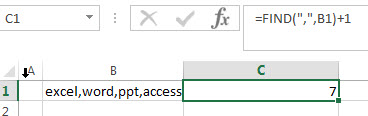The FIND function returns the position of the first comma character in Cell B1. It returns 6. And then add 1 to get the position of the first character after comma character. So this formula returns 7. The returned value goes into the MID function as its start_num argument.

=MID(B1,FIND(“,”,B1)+1,LEN(B1))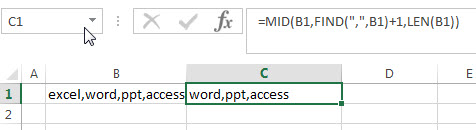So far, you got the values of the start_num and num_chars arguments from above FIND and LEN formula. And then the MID function extracts a substring based on the starting position and the number of the characters that you want to extract from a text string in Cell B1.

=SUBSTITUTE(B1,MID(B1,FIND(“,”,B1)+1,LEN(B1)),”excelhow”,1)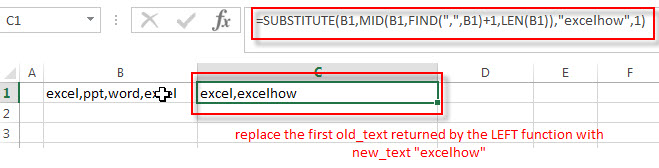This formula will replace the first old_text returned by the LEFT function with new_text “excelhow” in a text string in Cell B1.

## Replace all characters before a specific character using FIND&Select command

You can also use the Find and Replace command to replace all characters after a specified character, just refer to the following steps:

1# Click “HOME“->”Find&Select”->”Replace…”, then the window of the Find and Replace will appear.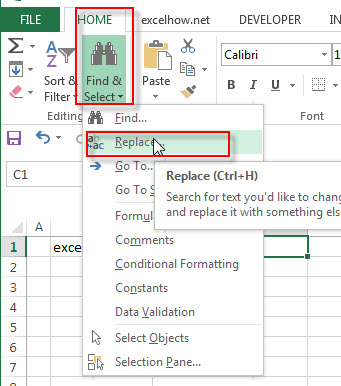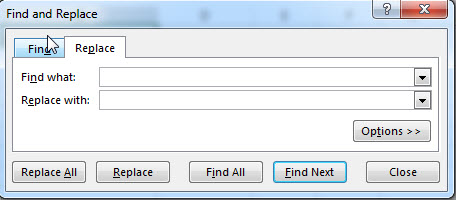2# click “Replace” Tab, then type ,* into the Find what: text box, and then type “,excelhow” string in the Replace with: text box.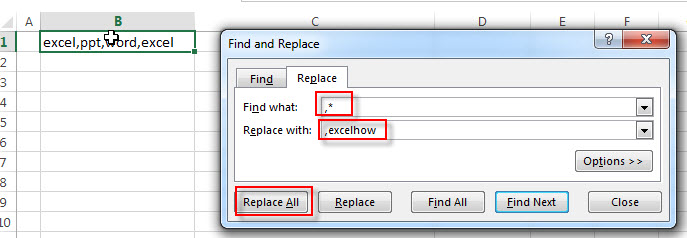3# click “Replace All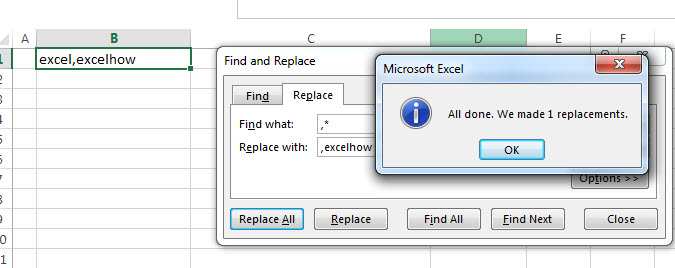you will see that all characters after the comma character are replaced with “excelhow” string.

### Related Functions

• Excel Substitute function
The Excel SUBSTITUTE function replaces a new text string for an old text string in a text string.The syntax of the SUBSTITUTE function is as below:= SUBSTITUTE  (text, old_text, new_text,[instance_num])….
• Excel MID function
The Excel MID function returns a substring from a text string at the position that you specify.The syntax of the MID function is as below:= MID (text, start_num, num_chars)….
• Excel FIND function
The Excel FIND function returns the position of the first text string (sub string) within another text string.The syntax of the FIND function is as below:= FIND(find_text, within_text,[start_num])…
• Excel SEARCH function
The Excel SEARCH function returns the number of the starting location of a substring in a text string.The syntax of the SEARCH function is as below:= SEARCH  (find_text, within_text,[start_num])…
• Excel LEN function
The Excel LEN function returns the length of a text string (the number of characters in a text string).The LEN function is a build-in function in Microsoft Excel and it is categorized as a Text Function.The syntax of the LEN function is as below:= LEN(text)…